# shock wave

(redirected from Shock Waves)
Also found in: Dictionary, Thesaurus, Medical, Acronyms.

## shock wave,

wave formed of a zone of extremely high pressure within a fluid, especially the atmosphere, that propagates through the fluid at a speed in excess of the speed of sound. A shock wave is caused by the sudden, violent disturbance of a fluid, such as that created by a powerful explosion or by the supersonic flow of the fluid over a solid object. Propagating from the point of the disturbance, a shock wave carries energy and can have destructive effects as it impinges on solid objects. A shock wave decays rapidly with increasing distance from its point of origin, gradually changing into an ordinary sound wave. Continuous shock waves, such as those produced by supersonic aircraft, are of particular concern as they tend to recur along regular routes. Even after they have decayed into sound waves, thus losing their destructive force, they remain capable of creating noise levels harmful to human beings and animals.

## Shock wave

A mechanical wave of large amplitude, propagating at supersonic velocity, across which pressure or stress, density, particle velocity, temperature, and related properties change in a nearly discontinuous manner. Unlike acoustic waves, shock waves are characterized by an amplitude-dependent wave velocity. Shock waves arise from sharp and violent disturbances generated from a lightning stroke, bomb blast, or other form of intense explosion, and from steady supersonic flow over bodies.

The abrupt nature of a shock wave in a gas can best be visualized from a schlieren photograph or shadow graph of supersonic flow over objects. Such photographs show well-defined surfaces in the flow field across which the density changes rapidly, in contrast to waves within the range of linear dynamic behavior of the fluid. Measurements of fluid density, pressure, and temperature across the surfaces show that these quantities always increase along the direction of flow, and that the rates of change are usually so rapid as to be beyond the spatial resolution of most instruments. These surfaces of abrupt change in fluid properties are called shock waves or shock fronts. See Schlieren photography

Shock waves in supersonic flow may be classified as normal or oblique according to whether the orientation of the surface of abrupt change is perpendicular or at an angle to the direction of flow. A schlieren photograph of a supersonic flow over a blunt object is shown in the illustration. Although this photograph was obtained from a supersonic flow over a stationary model in a shock tube, the general shape of the shock wave around the object is quite typical of those observed in a supersonic wind tunnel, or of similar objects (or projectiles) flying at supersonic speeds in a stationary atmosphere. The shock wave in this case assumes an approximately parabolic shape and is clearly detached from the blunt object. The central part of the wave, just in front of the object, may be considered an approximate model of the normal shock; the outer part of the wave is an oblique shock wave of gradually changing obliqueness and strength.

Some common examples of shock waves in condensed materials are encountered in the study of underground or underwater explosions, meteorite impacts, and ballistics problems. The field of shock waves in condensed materials (solids and liquids) has grown into an important interdisciplinary area of research involving condensed matter physics, geophysics, materials science, applied mechanics, and chemical physics. The nonlinear aspect of shock waves is an important area of applied mathematics.

Experimentally, shock waves are produced by rapidly imparting momentum over a large flat surface. This can be accomplished in many different ways: rapid deposition of radiation using electron or photon beams (lasers or x-rays), detonation of a high explosive in contact with the material, or high-speed impact of a plate on the sample surface. The impacting plate itself can be accelerated by using explosives, electrical discharge, underground nuclear explosions, and compressed gases. The use of compressed gas to accelerate projectiles with appropriate flyer plates provides the highest precision and control as well as convenience in laboratory experiments.

Large-amplitude one-dimensional compression and shear waves have been studied in solids. In these experiments, a macroscopic volume element is subjected to both a compression and shear deformation. The combined deformation state is produced by impacting two parallel flyer plates that are inclined at an angle to the direction of the plate motion. Momentum conservation coupled with different wave velocities for compression and shear waves leads to a separation of these waves in the sample interior. These experiments provide direct information about the shear response of shocked solids, and subject samples to more general loading states than the uniaxial strain state.

Shock waves subject matter to unusual conditions and therefore provide a good test of understanding of fundamental processes. The majority of the studies on condensed materials have concentrated on mechanical and thermodynamic properties. These are obtained from measurements of shock velocity, stress, and particle velocity in well-controlled experiments. Advanced techniques using electromagnetic gages, laser interferometry, piezoelectric gages, and piezoresistance gages have given continuous, time-resolved measurements at different sample thicknesses.

The study of residual effects, that is, the postshock examination of samples subjected to a known pulse amplitude and duration, is of considerable importance to materials science and metallurgy. The conversion of graphite to diamonds is noteworthy. Other effects that have been observed are microstructural changes, enhanced chemical activity, changes in material hardness and strength, and changes in electrical and magnetic properties. The generation of shock-induced lattice defects is thought to be important for explaining these changes in material properties. There has been growing interest in using shock methods for material synthesis and powder compaction.

## shock wave

(shock front) A discontinuity in the physical properties (pressure, temperature, density) of a gas, liquid, or solid that travels through the fluid or solid medium at supersonic speed without further change. Shock waves are generated, for example, by earthquakes, by explosions such as supernovae, or by impacts of supersonic bodies, as when the solar wind meets the Earth's magnetosphere. A shock wave can lose energy by heating the medium through which it travels.

## Shock Wave

a region characteristic of the supersonic flow of a gas. In this region, the velocity of the gas abruptly decreases, and a corresponding increase occurs in the pressure, temperature, density, and entropy. The thickness of a shock wave in the direction normal to its surface is the distance over which the change in the gas parameters occurs. The thickness of a shock wave in the direction normal to its surface is the distance over which the change in the gas parameters occurs. This thickness is small—of the order of the mean free path of the molecules—and is therefore neglected in most problems of gas dynamics.

## Shock Wave

a thin transition region that propagates at supersonic speed and in which the density, pressure, and speed of the substance involved increase drastically.

Shock waves result from, for example, explosions, the motion of bodies at supersonic speeds (seeSUPERSONIC FLOW), and intense electrical discharges. Thus, the detonation of an explosive causes the formation of highly heated explosion products of considerable density that are under high pressure. At the initial instant they are surrounded by air at rest at normal density and atmospheric pressure. The expanding explosion products compress the surrounding air. At each instant only the air located in a definite volume is compressed; outside this volume the air remains in the undisturbed state. The shock wave, or shock front, is the surface separating the compressed air from the undisturbed air.

The classical example of the development and propagation of shock waves is provided by the compression of gas in a tube by a piston. If the piston moves slowly into the gas, an acoustic (elastic)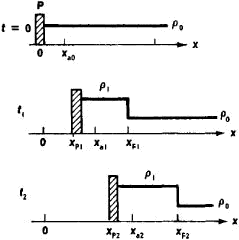Figure 1. Diagram of piston motion, density distribution, and position of shock front: (P) piston, (p) density, (F) shock front

compression wave travels through the gas at the speed of sound a. If, however, the speed of the piston is not small compared with the speed of sound, a shock wave arises. The rate of propagation of the shock wave through the undisturbed gas

vwave = (xF2xF1)/(t2t1)

(Figure 1) is greater than the speed of a particle of the gas; the particle speed is equal to the speed of the piston

v = (xP2xP1)(t2t1)

The distances between particles in the shock wave are less than in the undisturbed gas because of the compression of the gas. If the piston initially moves into the gas at a low speed and is gradually accelerated, the shock wave is not formed immediately. Instead, there first arises a compression wave, which exhibits a continuous distribution of density ρ and pressure p. With time, the front side of the compression wave becomes increasingly steep, since disturbances from the accelerating piston overtake the wave and intensify it. As a result, a sharp jump occurs in all hydrodynamic quantities—that is, a shock wave is formed.

Laws of shock compression. When a gas passes through a shock wave, the parameters of the gas change abruptly in a very narrow region. The thickness of the shock front is of the order of the mean free path of the molecules. In many theoretical studies, however, such a small thickness may be disregarded, and the shock front may be replaced with little loss of accuracy by a surface of discontinuity; the parameters of the gas are considered to change abruptly when the gas crosses the discontinuity. The relations between the values of the gas parameters on the two sides of the discontinuity are given by the following equations, which result from the laws of conservation of mass, momentum, and energy: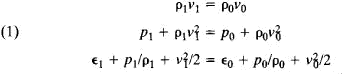Here, p1 is the pressure, p1 the density, ∊1 the specific internal energy, and v1 the speed (as measured in a coordinate system moving with the shock front) of the gas behind the shock front; p0, p0, ∊0, and v0 are the values of the same quantities ahead of the front. The speed v0 at which the gas flows into the discontinuity is equal to the rate of propagation vwave of the shock wave through the undisturbed gas.

By eliminating the speeds from equations (1), the equations of what is called the Hugoniot curve can be obtained: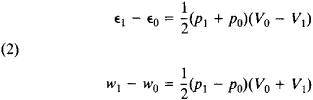where V = 1/ρ is the specific volume and w = ∊ + p/ρ is the specific enthalpy. If the thermodynamic properties of the gas, that is, the functions ∊(p,/ρ) or w (p, p), are known, then the Hugoniot curve gives the dependence of the final pressure p1 on the final volume V1 for shock compression of the gas from the given initial statep p0, V0. Thus, we have the relation

P1 = H (V1, p0, V0)

When a gas passes through a shock wave, the entropy S of the gas changes. The entropy jump S1S0 for a given gas is determined solely by the conservation laws [equations (1)], which allow the existence of both a compression wave (ρ1 > ρ0 and p > p0) and a rarefaction wave (ρ1 < ρ0 and p1 < p0). The second law of thermodynamics, however, requires that entropy increase. In ordinary substances the entropy increases only in a compression wave, and the rarefaction shock wave is therefore not realized (Zemplen’s theorem).

A shock wave propagates through an undisturbed gas at the supersonic speed v0 > a0, where a0 is the speed of sound in the undisturbed gas. The value of v0 increases with the strength of the shock wave, the strength being defined here as (p1p0)/p0. As the strength of the shock wave approaches zero, the speed of propagation approaches a0. The speed of a shock wave with respect to the compressed gas behind it is subsonic: V1 < a1, where a1 is the speed of sound in the compressed gas behind the shock wave.

Shock waves in an ideal gas of constant specific heats. The simplest case of propagation of a shock wave is that where the shock occurs in an ideal gas of constant specific heats. The equation of state in this case has an extremely simple form:

∊ = p/ρ(γ – 1)

P = RρT

where γ = Cp/Cv is the ratio of the specific heats at constant pressure and volume (sometimes called the isentropic exponent), R is the universal gas constant, and u, is the molecular weight. The equation of the Hugoniot curve can then be obtained in the explicit form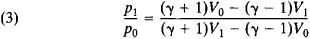The Hugoniot curve H differs from the ordinary isentrope P, for which p1/p0 = (V0/V1)γ (Figure 2). For a given change in V, shock compression of the gas requires a greater change in p than does adiabatic compression. This fact is a consequence of the irreversibility of the heating during shock compression; the heating is due to the conversion into heat of the kinetic energy of the flow incident on the shock front. It follows from equations (1) that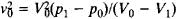Consequently, the speed of the shock wave is determined by the slope of the line joining the points of the initial and final states (Figure 2).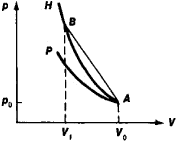Figure 2. Hugoniot curve (H) and ordinary isentrope (P) passing through a common initial point (A) of the initial state

The parameters of a gas in a shock wave may be represented as a function of the Mach number M = vwave/a0: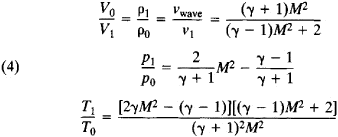In the limit, as M → ∞ and P1\p0, → ∞ we obtain for strong shock waves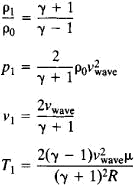Thus, an arbitrarily strong shock wave cannot compress a gas by a factor greater than (γ + l)/(γ – 1). In the case of a monatomic gas, for example, γ = 5/3, and the limiting factor is 4; in the case of a diatomic gas (air) γ = 7/5, and the limiting factor is 6. The higher the specific heat of the gas (the smaller γ), the higher the limiting factor.

Viscosity and heat conduction. The irreversibility of shock compression indicates that mechanical energy is dissipated in the shock front. Dissipative processes can be taken into account by allowing for the viscosity and thermal conductivity of the gas. Here it turns out that the entropy change across the shock wave depends neither on the mechanism of dissipation nor on the gas’s viscosity and thermal conductivity, which determine only the internal structure of the wave front and its thickness. In a shock wave of moderate strength, the quantities v, p, p, and T change monotonically from their initial values to their final values (Figure 3). By contast, the entropy 5 does not change monotonically and within a shock wave reaches a maximum at the point of inflection of the speed curve, that is, at the center of the wave. The occurrence of the maximum of S in the wave is due to the existence of heat conduction. Viscosity leads only to an increase in entropy: as a result of viscosity, some of the momentum of the ordered gas flow incident on the shock wave is dissipated, the kinetic energy of ordered molecular motion being converted into the energy of random motion, that is, into heat. As a result of heat conduction, heat is transferred irreversibly from hotter layers of the gas to less hot layers.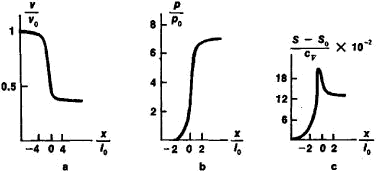Figure 3. Distribution of three parameters across a shock wave with the Mach number M = 2 in a gas, in the presence of viscosity and heat conduction: (a) speed, (b) pressure, (c) entropy

Shock waves in real gases. In a real gas at high temperatures a number of processes occur—such as the excitation of molecular vibrations, the dissociation of molecules, chemical reactions, and ionization—that involve the expenditure of energy and a change in the number of particles. Here, the internal energy e depends in a complicated manner on p and ρ, and the parameters of the gas behind the shock front can be determined only through numerical calculations using equations (1) and (2).

A very great number of molecular collisions are usually required in order to redistribute among different degrees of freedom the energy of a gas compressed and heated in a strong shock wave. In real gases the width Ax of the layer across which the transition from the initial to the final states of thermodynamic equilibrium occurs, that is, the width of the shock front, there-fore is usually much greater than the width of the “viscous” wave (that is, the wave as determined by the viscosity mechanism). The quantity Δx is determined by the relaxation time of the slowest processes, such as the excitation of vibrations, dissociation, and ionization. The temperature and density distributions across the shock wave have the form illustrated in Figure 4, where the viscous shock wave is represented as a discontinuity.

If the gas behind the front of a shock wave is strongly ionized or if the shock wave propagates in a plasma, the ion and electron temperatures in the shock wave do not coincide. Heavy particles, but not electrons, are heated in the shock wave, and the energy exchange between ions and electrons occurs slowly because of the great difference in their masses. Relaxation involves the equalization of temperatures. Moreover, an important role in the propagation of shock waves in a plasma is played by the electronic thermal conductivity, which is much greater than the ionic thermal conductivity and which leads to the heating of electrons in front of the shock wave. Magnetohydrodynamic shock waves propagate in an electrically conducting medium in the presence of an external magnetic field. The theory of such waves is constructed, on the basis of the equations of magnetohydrodynamics, in much the same way as the theory of ordinary shock waves.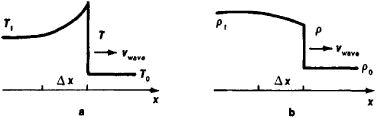Figure 4. Distribution of temperature and density across a shock wave propagating in a real gas: (a) temperature distribution, (b) density distribution

At temperatures above a few tens of thousands of degrees the structure of shock waves is influenced considerably by radiative heat transfer. The mean free paths of light quanta are usually much greater than the gas-kinetic paths, and it is the mean free paths of light quanta that determine the thickness of the front. All gases are opaque in the far-ultraviolet region of the spectrum; the high-temperature radiation emerging from behind the shock wave is therefore absorbed ahead of the wave and heats the uncompressed gas. Behind the wave the gas is cooled through losses to radiation. In this case the width of the front is of the order of the mean free path of the radiation, which is 10–2–10–1 cm in air of normal density. The higher the temperature behind the front, the larger the radiant flux from the surface of the wave and the higher the temperature of the gas ahead of the wave. The hot gas ahead of the wave does not transmit the visible light coming from behind the front and thus screens the front. The brightness temperature of shock waves therefore does not always coincide with the true temperature behind the front.

Shock waves in solids. The energy and pressure in solids have a dual nature: they are due both to the thermal motion and the interaction of particles—that is, they have both thermal and elastic components. The theory of interparticle forces does not give the general density dependence of the elastic components of the pressure and energy over a broad range for different substances, and the function ∊(p/ρ) therefore cannot be constructed theoretically. The Hugoniot curves for solids (and liquids) are consequently determined experimentally or semiempirically. Pressures of millions of atmospheres, which have been attained in experimental research, are required for significant compression of solids. In practice, weak shock waves with pressures of 104–105 atmospheres are of great importance. Such pressures are developed, for example, in a detonation, in explosions in water, and in impacts of explosion products on barriers. The increase in entropy in shock waves with such pressures is not great, and the shock wave propagation is usually calculated by means of an empirical equation of state of the type p = A [(ρ/ρ0)n – 1], where the quantity A, which in general depends on the entropy, is, like n, regarded as a constant. Shock waves cause polymorphic transitions in a number of substances, such as iron and bismuth. At moderate pressures elastic waves occur in solids. The propagation of such waves, like that of weak compression waves in gases, may be studied on the basis of the laws of acoustics.

### REFERENCES

Landau, L. D., and E. M. Lifshits. Mekhanika sploshnykh sred, 2nd ed. Moscow, 1953.
Zel’dovich, Ia. B., and Iu. P. Raizer. Fizika udarnykh voln i vysokotemperaturnykh gidrodinamicheskikh iavlenii, 2nd ed. Moscow, 1966.
Stupochenko, E. V., S. A. Losev, and A. I. Osipov. Relaksatsionnye protsessy v udarnykh volnakh. Moscow, 1965.

IU. P. RAIZER

## shock wave

[′shäk ‚wāv]
(physics)
A fully developed compression wave of large amplitude, across which density, pressure, and particle velocity change drastically.

## shock wave

An area or sheet of discontinuity (i.e., of abrupt changes in conditions) set up in a supersonic field of flow, through which the fluid undergoes a finite decrease in velocity accompanied by a marked increase in pressure, density, temperature, and entropy, as occurs in a supersonic flow about a body. The various types of shock waves include attached shock wave, bow wave, detached shock wave, Mach wave, normal shock wave, and oblique shock wave. Sometimes called a shock. See attached shock wave, detached shock wave, normal shock, and oblique shock wave.
References in periodicals archive ?
Contemporary clinical practice of shock wave lithotripsy: A reevaluation of contraindications.
Treatment of lateral epicondylitis of the elbow with shock waves. Clin Orthop Relat Res.
Under such circumstances, the paper conducted researches on the most common one-way bifurcation pipeline and adopted the shock wave attenuation law under laboratory one-way bifurcation pipeline to simulate shock wave attenuation law under the practical one-way bifurcation pipeline.
The tester took liquid as storage medium to simulate the shock wave based on controlled hydraulic technology.
According to the company, the claimed methods of the patent use shock waves generated via plasma bubbles created with laser emitters.
where P is the peak pressure of the shock wave, Pa; [beta]is a complex integration, the value of which is 0.7 in water, dimensionless; [rho] is the water density, kg/[m.sup.3]; [tau] is the leading edge of the shock wave, s; T is the bottom width of the shock wave, s; w is the total energy of the discharge current per unit length of the discharge channel, J/m; k is the coefficient, dimensionless; C is the electric capacity, F; U is the voltage, kV; r is the spreading distance of the shock wave, m.
Across a shock wave, the static pressure, temperature, and gas density increases enormously.
When the sphere is kept stationary throughout its collision with the oncoming shock wave, the drag coefficient is directly deduced from the computed pressure distribution around the sphere.
In other words, we assume that the Riemann solution starting from the origin (0, 0) is always two shock waves. In this situation, we will construct the global solutions of the perturbed Riemann problem (1) and (2) case by case by investigating the wave interaction problems in detail.
Tsykanovskii, "Seismic, hydroacoustic, and acoustic action of underwater explosions," Combustion, Explosion and Shock Waves, vol.
To study the perturbation of a shock wave in conservation laws with physical viscosity, Liu and Zeng obtain the detailed pointwise estimates of the solutions, and show that the solution converges to a translated shock profile.
She underwent SWL using 40 shock waves per minute with electrohydraulic shock wave lithotripter.

Site: Follow: Share:
Open / Close